# Seismic AVO analysis of methane hydrate structures

Christine Ecker and David E. Lumley

Author has no known email address

# ABSTRACT

Marine seismic data from the Blake Outer Ridge offshore Florida show strong ``bottom simulating reflections'' (BSR) associated with methane hydrate occurence in deep marine sediments. We use a detailed amplitude versus offset (AVO) analysis of these data to explore the validity of models which might explain the origin of the bottom simulating reflector. After careful preprocessing steps, we determine a BSR model which can successfully reproduce the observed AVO responses. The P- and S-velocity behavior predicted by the forward modeling is further investigated by estimating the P- and S-impedance contrasts at all subsurface positions. Our results indicate that the Blake Outer Ridge BSR is compatible with a model of methane hydrate in sediment, overlaying a layer of free methane gas-saturated sediment. The hydrate-bearing sediments seem to be characterized by a high P-wave velocity of approximately 2.5 km/s, an anomalously low S-wave velocity of approximately 0.5 km/s, and a thickness of around 190 meters. The underlaying gas-saturated sediments have a P-wave velocity of 1.6 km/s, an S-wave velocity of 1.1 km/s, and a thickness of approximately 250 meters.

Bottom simulating reflectors, so called BSRs, parallel the seafloor at subbottom depths of several hundred meters. Seismic investigations (, , ) indicate that they are characterized by large negative reflection coefficients and increasing subbottom depth with increasing water depth. The base of the stability field for methane hydrates appears to be associated with these bottom simulating reflectors. Due to the enormous amount of methane that is apparently contained within hydrate structures, they are likely to have a significant ``greenhouse'' effect on future global climate, and might also represent an important future energy resource (). Therefore, a good understanding of the origin and characteristics of the hydrate zones and BSRs is desirable. Only limited information is available from deep-sea drilling, as the risk of heating and destabilizing the initial hydrate conditions during the process of drilling is considerably high. Thus, the core samples and well-logs do not necessarily reflect the correct in situ hydrate characteristics and properties. Consequently, most information is inferred remotely from seismic reflection data (, , , , ). Most of these investigations, which were based mainly on AVO responses and synthetic modeling, used primarily P-velocity information, accessible directly from the seismic data, and neglected possible important S-velocity effects entirely. The exact formation of the hydrate and its formation are still controversial, and different models have been proposed to explain the origin of the BSRs (, ).

In this study, we use both P- and S-wave information inferred from synthetic modeling, velocity and AVO analysis of marine data from the Blake Outer Ridge to explain the bottom simulating reflector. The validity of the different BSR models is explored and the effects of two proposed models can be clearly discriminated. The reflection amplitude variation with offset can be an important indicator of free gas at an interface () and, together with the estimation of material properties at the interface, considerably limits the possible explanations of the physical origin of the BSR.

This paper discusses our work with preprocessing, modeling, inversion, and interpretation of the methane hydrate seismic data from the Blake Outer Ridge. Preliminary results of this study were presented by Ecker and Lumley . After careful preprocessing, including source wavelet deconvolution, trace interpolation, and amplitude and moveout traveltime calibration, a detailed velocity analysis is performed on the resulting CMP gathers. The estimated interval velocities are used to constrain a BSR model which can successfully reproduce the observed AVO amplitude responses. The impedance structure predicted by the modeled data is further reinforced by estimating the P- and S-impedance contrasts at all subsurface positions. Combining the results of the synthetic modeling and the impedance inversion, we give an integrated geophysical interpretation of the data.

METHANE HYDRATES AND BSR MODELS

A methane hydrate is an ice-like, crystalline lattice of water molecules in which gas molecules are trapped physically without the aid of direct chemical bonds. They are stable under certain pressure and temperature conditions (Figure phase). Thus, the occurrence of bottom simulating reflectors is restricted to two distinct regions: deep oceanic and polar. In deep oceanic regions, BSRs are found in outer continental margins of slopes and rises where cold bottom water is present. In polar regions, the BSRs are normally associated with permafrost, both onshore in continental sediments and offshore in sediments of the continental shelves.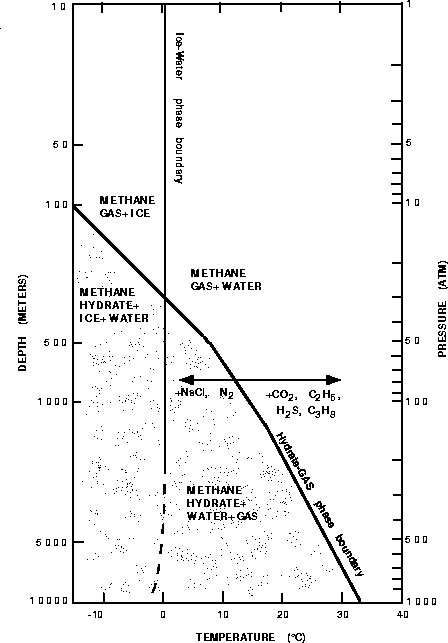phase
Figure 1
Phase diagram showing the boundary between methane gas and methane hydrate. Redrawn after Kvenvolden (1993).Two models have been proposed to account for gas hydrate formation and thus the development of BSRs. The first one assumes the local generation of methane from organic material at the depth of the hydrate. Gradually thickening and thus deepening of the methane hydrate zone causes it eventually to subside into a temperature region where hydrate is unstable. Consequently, free gas can be present in this region (). The BSR is caused by the impedance contrast at the base of the hydrate layer and the top of the gas layer. A second model, on the other hand, supports the formation of methane hydrates through the removal of methane from rising pore fluids being expelled upwards from deeper in the sediment section (). Most of the methane is generated microbially at depths below the hydrate stability zone but not at depths sufficient for the formation of thermogenic methane. Thus free gas does not have to be present below the BSR. In this case, the BSR can be the consequence of the impedance contrast between overlaying sediments containing substantial amounts of high-velocity hydrate and underlaying normal velocity brine sediments.

PREPROCESSING STEPS

In the first preprocessing step, the data were corrected for time-varying spherical divergence. Next, we performed a single-trace source wavelet deconvolution in order to regularize the source wavelet with offset. The deconvolved data were then bandpass filtered to the original data bandwidth to remove spurious deconvolutional high-frequency noise. Using an initial semblance estimate of the best stacking velocity function, a normal moveout correction of the data was carried out.

Two main assumptions were made to perform the amplitude calibration. First, it was assumed that an offset-dependent rather than an angle-dependent amplitude correction was sufficient, since the difference between the maximal angle of incidence at the seafloor (30) and the BSR reflection (33) is negligibly small. Second, we assumed a functional form for the AVO response of the seafloor reflection, based on the fluid-solid interface Zoeppritz PP reflection coefficient. Based on these assumptions, the amplitude calibration was performed by scaling each trace to the seabottom amplitude to match the predicted seafloor AVO as a function of offset.

Due to the use of a nonlinear streamer to record the data, a trace interpolation of the near offset data was necessary to regularize the receiver cable group spacings. After applying an inverse NMO correction to the interpolated data, a high resolution NMO stacking velocity analysis was performed. Having derived a good stacking model for the data, they were reprocessed in a second iteration using the new velocities. Since it is essential for the subsequent impedance contrast estimation that the reflector moveout is very flat after NMO correction or migration, an additional static shift was applied preceding the amplitude scaling to correct for some small non-hyperbolic, offset-dependent residual moveout in the CMP gathers.

Figure bsr-ann shows the final data after preprocessing. The gather contains a BSR AVO effect that is representative of the average trend along the entire line. Picking the peak amplitudes along the BSR yielded the AVO curve shown in Figure bsr-picks. Starting with a negative zero-offset reflection coefficient that was obtained by assuming a seafloor reflection of approximately 0.2, the amplitudes become increasingly negative with increasing offset.bsr-ann
Figure 2
Final CMP gather after preprocessing containing an average AVO effect observed along the line.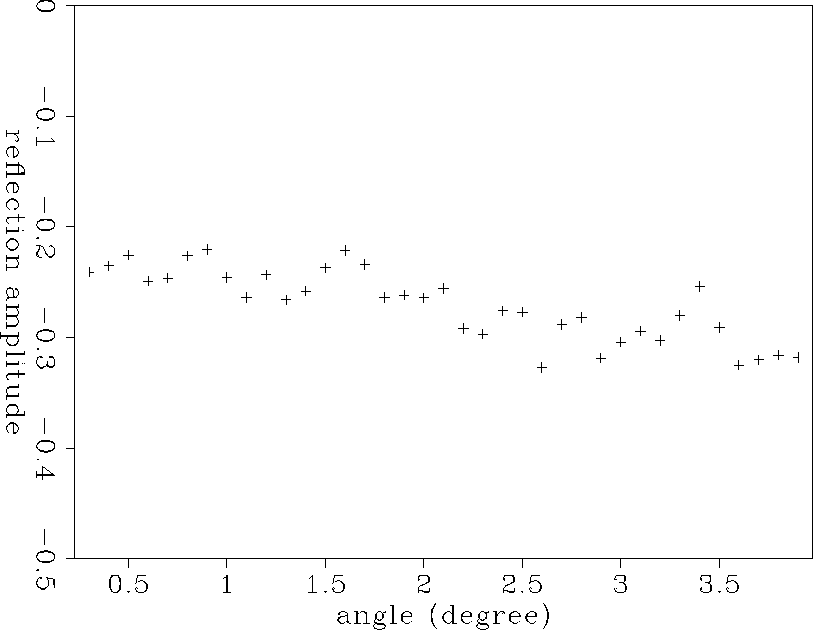bsr-picks
Figure 3
AVO curve of the amplitude picks along the BSR. The near offset reflection amplitude was determined by assuming a seafloor reflection of approximately 0.2.MODELING APPROACH

Using the estimated interval velocities, the effects of different impedance structures on the BSR AVO response were explored in an attempt to reproduce the seismic data. Several models were constructed which were constrained to preserve the average interval velocity of each macro layer. To avoid possible tuning effects in this first, basic modeling approach, all layers were assumed to be thicker than a quarter of a wavelength. Synthetic AVO amplitude responses were then estimated for the individual models using Zoeppritz equations and compared with the amplitude responses observed in the seismic data.

Figure orig shows the initial P- and S-wave interval velocities. The P-wave velocity was inferred directly from the seismic data, while the S-wave velocity was determined by assuming a Poisson's ratio of 0.4 which is consistent with brine saturated sediments.orig
Figure 4
Initial velocity model of the data.A considerably high P-wave velocity of approximately 2.5 km/s was obtained above the BSR which appears to be underlain by a lower velocity of around 1.6 km/s. The P-velocity trend for normal brine-saturated sediments is indicated by the dotted line. Being higher than this trend above the BSR and lower below it, the measured P-wave velocities might be compatible with a model of hydrate-bearing sediment overlaying gas-saturated sediments.

In a first attempt to model the observed AVO amplitudes, a thin layer of high-velocity, hydrate-bearing sediments was assumed to overlay brine-saturated sediments. As the measured P-wave interval velocity of 2.5 km/s above the BSR has to be preserved, the hydrate layer can not be smaller than a certain thickness in order to obtain realistic velocity values for this model. The modeled P- and S-wave velocities above and below the BSR are shown in Figure thin-hydrate. The initial model is given by the dotted line whenever the modeled velocities differ from the initial ones. The S-wave velocity was obtained by assuming that the Poisson's ratio in the hydrate-bearing sediments is comparable with that of brine sediments.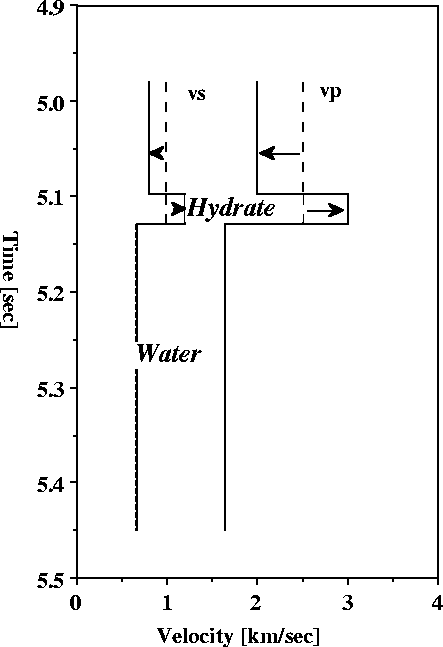thin-hydrate
Figure 5
Interval velocities above and below the BSR for a thin-hydrate layer overlaying brine sediment. The dotted lines represent the velocities of the initial model, while the solid lines give the velocities of this model. The arrows indicate the direction of the velocity change.Using Zoeppritz equations, the AVO trend corresponding to the modeled velocities at the transition from hydrate to brine-saturated sediments is determined and compared with the one observed in the data (Figure thin-hyd).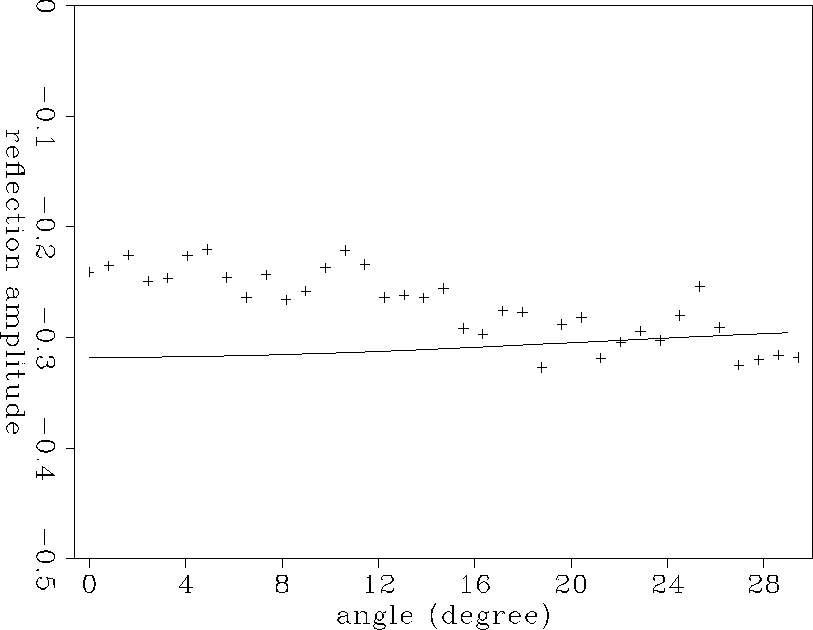thin-hyd
Figure 6
AVO Curve obtained from the thin-hydrate model (solid line) compared with the one observed in the data (crosses).A comparison of both curves yields that the thin-hydrate model not only failed in reproducing the near offset reflection coefficients, but also the general AVO trend, having slightly increasing amplitudes with increasing offset. Assuming negligibly small density contributions, the near offset amplitudes are mainly dependent on the P-wave velocity contrast at the reflector, while the AVO trend is characterized primarily by the S-wave velocity contrast. Thus, the AVO response resulting from the thin-hydrate model implies the use of both incorrect P- and S-wave velocities at the bottom simulating reflector. Further thinning of the hydrate layer would increase the P-wave velocity in this zone due to the required preservation of the measured interval velocity, and thus the P-wave velocity contrast at the BSR. This would result in an even more pronounced difference between the observed and modeled zero offset reflection amplitudes. Consequently, a thin-hydrate layer overlaying brine-saturated sediments is not sufficient to explain the seismic data.

Based on this result, the subsequent modeling attempted to decrease the P-wave velocity contrast at the BSR in order to recreate the observed zero offset reflection amplitudes. The required decrease was performed by thickening the hydrate layer, thus yielding a thick-hydrate over brine sediment model. An evaluation of the effects of several different velocity combinations on the reflection amplitudes resulted in the model shown in Figure brine-vel. The estimated P-wave velocity in the hydrate corresponds to the measured interval velocity, yielding a considerable thickness of the hydrate zone. The S-wave velocity was again obtained using a Poisson's ratio of 0.4 and is thus the same as in the initial model. The AVO curve based on the transition from the hydrate to the brine-saturated sediments was determined by Zoeppritz modeling and is shown in Figure brine.

The comparison of the modeled AVO responses with those observed indicates that this model could successfully reproduce the zero offset data. This suggests that the modeled P-wave velocities of 2.5 km/s in the hydrate and 1.6 km/s in the underlaying sediments might resemble the actual conditions at the BSR. However, the obtained AVO trend is still contrary to the observed one, displaying increasingly positive amplitudes with increasing offset. Hence, a change in Poisson's ratio seems to be required at the transition from the hydrate-bearing sediments above the BSR to the sediments below the BSR.

Continuously changing the possible velocities in the hydrate zone and the characteristics of the underlaying sediments resulted finally in a hydrate layer characterized by a P-wave velocity of approximately 2.5 km/s and an anomalously low S-wave velocity of around 0.5 km/s yielding a Poisson's ratio of 0.47. The hydrates appear to be underlain by sediments having a P-wave velocity of 1.6 km/s and an S-wave velocity of 1.1 km/s, yielding a Poisson's ratio of 0.1 which is consistent with free gas. The final model can be seen in Figure gas-vel. The initial model is given by the dotted line whenever the modeled velocities differ from the initial ones. Based on the determined interval velocities, the thickness of the hydrate layer was calculated to be approximately 190 meters and the one of the gas layer to be around 250 meters. Neglecting possible tuning effects, a thin gas layer was not a good model representation, as it required a decrease in P-wave velocity with respect to the hydrate layer to preserve the measured interval velocity below the BSR. Thus, it resulted in a significant deviation of the zero offset reflection amplitudes of the model and the true seismic data.

A comparison of the synthetic AVO curve obtained for the model shown in Figure gas-vel with the amplitude picks obtained from a representative CMP gather is shown in Figure gas. Both the synthetic and the real data AVO amplitude responses are in good agreement for near and far offsets. Thus, a significant increase in S-wave velocity and a simultaneous decrease in P-wave velocity at the transition from hydrate-bearing sediments to sediments containing free gas is required to explain the observed seismic data.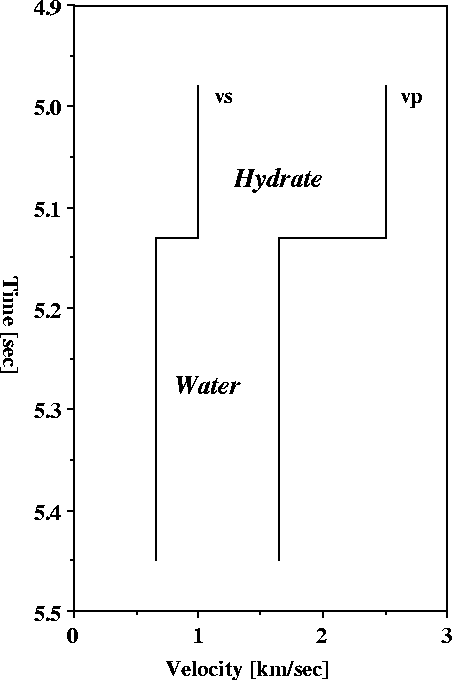brine-vel
Figure 7
Interval velocities above and below the BSR for a thick-hydrate over brine sediment model. The modeled velocities are correspond to the initial interval velocities.brine
Figure 8
AVO curve obtained from the thick-hydrate model (solid line) compared with the one observed in the data (crosses).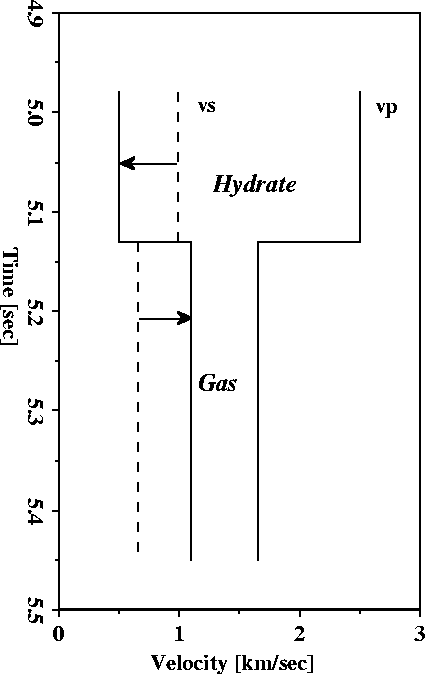gas-vel
Figure 9
Interval velocities for hydrate-bearing sediments overlaying gas-saturated sediments. The dotted lines represent the initial velocities. The arrows indicate where the modeled velocities had to be increased or decreased to match the seismic data.gas
Figure 10
Synthetic AVO curve of hydrates overlaying sediments saturated with free gas (solid line) compared with an observed one (crosses).The final velocity model for the entire section is shown in Figure final. The deviation from the initial interval velocities is indicated by the dotted line. While the initial P-wave interval velocities corresponded to the modeled velocities in the hydrate and gas sediments, the S-wave velocities had to be modified with regard to apparently different shear properties in the hydrates and the gas compared to the brine sediments.

Based on the modeled increase of the S-wave velocity at the bottom of the of the hydrate stability zone, a large positive S-impedance contrast can be predicted for the seismic data. On the other hand, a negative P-impedance contrast can be expected at the BSR due to the decrease in P-wave velocity at the transition from hydrate to gas. In order to determine the actual effect, we performed a prestack migration impedance inversion of the seismic data.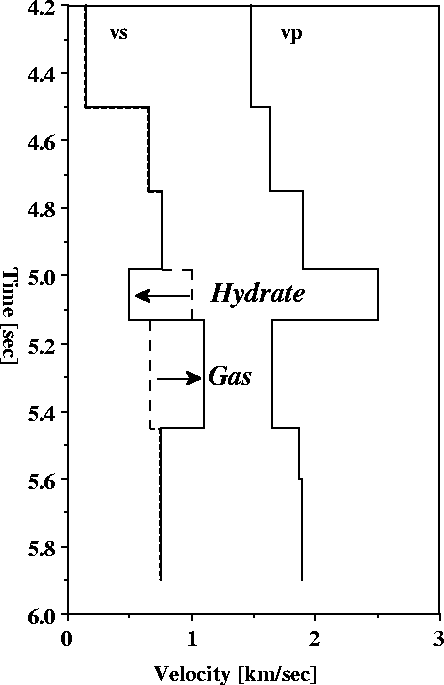final
Figure 11
Final modeled interval velocity model. The dotted line indicates where the initial model differs from the final model. The arrows describe the direction the velocity had to be changed in order to fit the seismic data.IMPEDANCE ESTIMATION

The P- and S-impedance contrasts at each subsurface position were estimated by applying a least-squares elastic parameter inversion method (, ) to the CMP gathers. This technique fits the prestack migrated AVO gathers at each pseudo-depth and surface position to the theoretical P- and S-impedance curves which are based on linearized Zoeppritz equations. The least-squares impedance inversion results of the preprocessed CMP gathers are shown in Figures pimp-ann and simp-ann.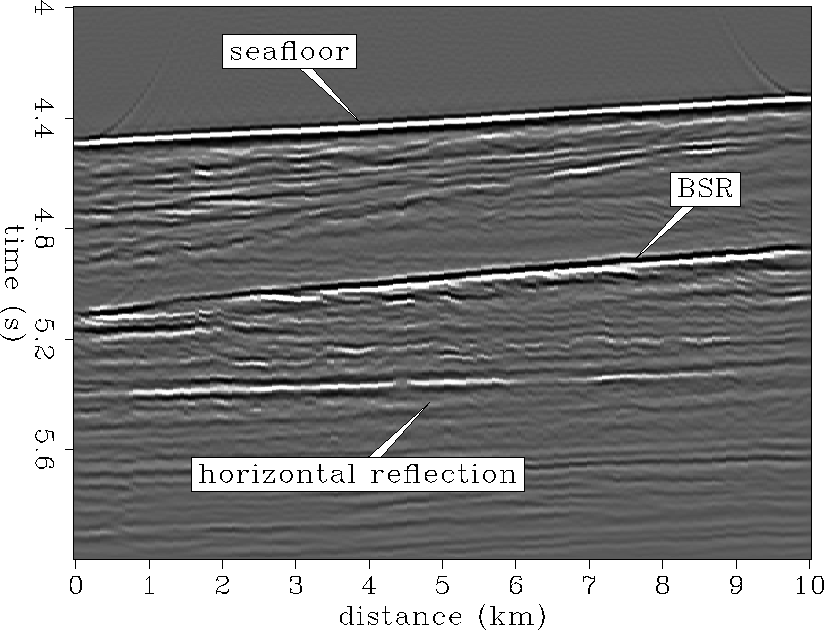pimp-ann
Figure 12
P-impedance contrast section.simp-ann
Figure 13
S-impedance contrast section.The P-impedance contrast section shows clearly that the seafloor reflection and the BSR have P-impedance contrasts of opposite polarity and approximately the same magnitude. In a small section above the BSR there is a ``quiet'' zone where no diffractions or reflections are visible, which can possibly be explained by the presence of disseminated methane hydrate. The S-impedance section is dominated by a very strong impedance contrast at the BSR. Although the seafloor has a much weaker impedance contrast, it is evident that both have the same polarity. Below the BSR, a horizontal reflector gives a strong P-impedance contrast of the same polarity as the seafloor, indicating an increase in P-wave velocity at the reflector, but weak S-impedance. This may be indicative of the base of the gas zone.

Assuming a seafloor reflection of 0.2 and assuming the P- and S-impedance contrasts at the seafloor, it is possible to estimate the relative impedance contrasts of the BSR by determining the average amplitude changes between seafloor and BSR. This results in a P-impedance contrast of -0.4 at the BSR which has the same magnitude but opposite polarity to the seafloor. The S-impedance contrast is very strong and amounts to approximately 0.8 to 1.2, which is two to three times as much as the seafloor impedance contrast of the same polarity. This anomalous S-impedance behavior is even more pronounced by making a simple P*S anomaly map shown in Figure PSmap-ann. Normal impedance structure is plotted dark grey, while anomalous impedance structure is indicated by white. The high magnitude contrast has the effect of visually diminishing the S-impedance contrast of the seafloor (Figure simp-ann) compared with the P-impedance contrast (figure pimp-ann) at the seafloor, which are actually the same magnitude.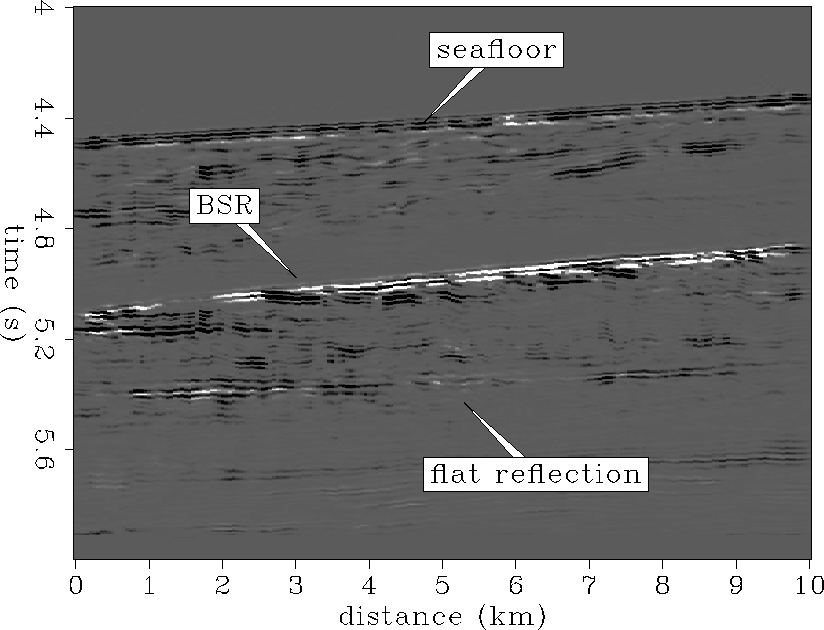PSmap-ann
Figure 14
P*S impedance contrast.The negative P-impedance contrast and the large positive S-impedance contrast at the BSR are in good agreement with the prediction based on the Zoeppritz modeling. The strong positive S-impedance contrast clearly supports the modeled S-wave velocity behavior of anomalously low S-velocity in the hydrates and considerably increased S-velocity in the underlain gas sediments. Based on the synthetic modeling and the impedance inversion results, the geology was interpreted as seen in Figure interp. In this interpretation, the hydrate-bearing sediments are assumed to overlay sediments in which free gas is trapped. The flat reflector below the BSR might correspond to a gas-water contact at the base of the free gas zone based on the impedance contrasts obtained for this reflector.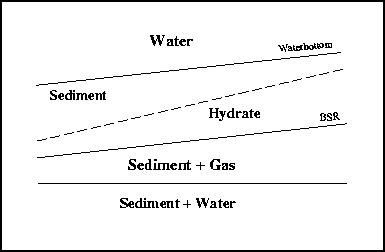interp
Figure 15
Interpretation of the methane hydrate seismic data from the Blake Outer Ridge.CONCLUSIONS

A detailed AVO analysis was performed on data from the Blake Outer Ridge to evaluate the origin of the bottom simulating reflector. Reflectivity clearly discriminates the effects of different models and shows that the observed BSR best fits a model of sediments containing substantial amounts of hydrate overlaying sediments containing free gas. This modeling result was supported by a prestack impedance inversion of the seismic data. A transition from hydrate to brine sediments is not consistent with the AVO amplitude responses and the impedance contrasts.

Based on the synthetic modeling, the thickness of the hydrate layer was determined to be approximately 190 meters. It is characterized by a P-wave velocity of around 2.5 km/s and an anomalously low S-wave velocity of 0.5 km/s. The thickness of the gas layer was calculated to be approximately 250 meters. It has a P-wave velocity of 1.6 km/s and an S-wave velocity of 1.1 km/s, yielding a Poisson's ratio of 0.1 which is reasonable for gas. The considerable thickness of the gas layer might suggest the possibility of it being a source rock for the overlaying methane hydrate.

It has to be considered, however, that the synthetic modeling excluded possible tuning effects and thus might represent a simplification of the actual conditions. Nevertheless, the velocity behavior predicted by the model was reinforced by the prestack impedance inversion, thus indicating that a transition from high P-wave velocity and anomalously low S-wave velocity in the hydrate to low P-wave velocity and high S-wave velocity in the gas sediments is required. A detailed investigation of this unusual behavior is performed by Ecker .

We thank the sponsors of the Stanford Exploration Project and Professor Jon Claerbout for supporting this research. We also thank Keith Kvenvolden, Bill Dillon and Myung Lee of the USGS for providing us with a copy of the Blake Outer Ridge seismic data used in this study.

[MISC,SEP]